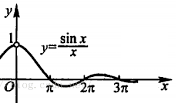# 极限定义新讲：动态定义与静态定义

$$${|a}_{n_{1}} - L\left| > {|a}_{n_{2}} - L \right| > {|a}_{n_{3}} - L| > \ldots$$$1. 总有后面的项比前面的项更接近于实数L，对应的数学语言描述是：数列 $$${\{ a}_{n}\}$$$ 中存在 $$$a_{n_{1}}$$$ $$$a_{n_{2}}$$$ $$$a_{n_{3}}$$$ ，…满足 $$${|a}_{n_{1}} - L| \geq {|a}_{n_{2}} - L| \geq {|a}_{n_{3}} - L| \geq \ldots$$$ ，其中 $$$n_{1},\ n_{2},n_{3},\ldots$$$ 均是原数列中满足本不等式的任意一项的下标，且 $$$n_{1} < n_{2} < n_{3} < \ldots$$$

2. 足够靠后的所有项可以接近实数L到任意程度，对应的数学语言描述是：总有某项之后的所有 $$$a_{n}$$$ 满足 $$${|a}_{n} - L| < \frac{1}{10^{M}}$$$ ，此处M是预先任意指定的正整数，这里的“某项”只能根据数列的表达式解这个不等式来确定。

1. 数学分析，华东师范大学数学系编，第四版，p23 ↩︎

2. What Is Mathematics? Second Edition, Courant and Robbins, p306 ↩︎

3. 说它“熟悉”是因为它是教材中常用的定义，说它“陌生”是因为这个定义不那么直观，不能反映极限的“动态性”。 ↩︎

4. Richard Courant, Fritz John, Introduction to Calculus and Analysis Volume I, Reprint of the 1989 edition, p82 ↩︎